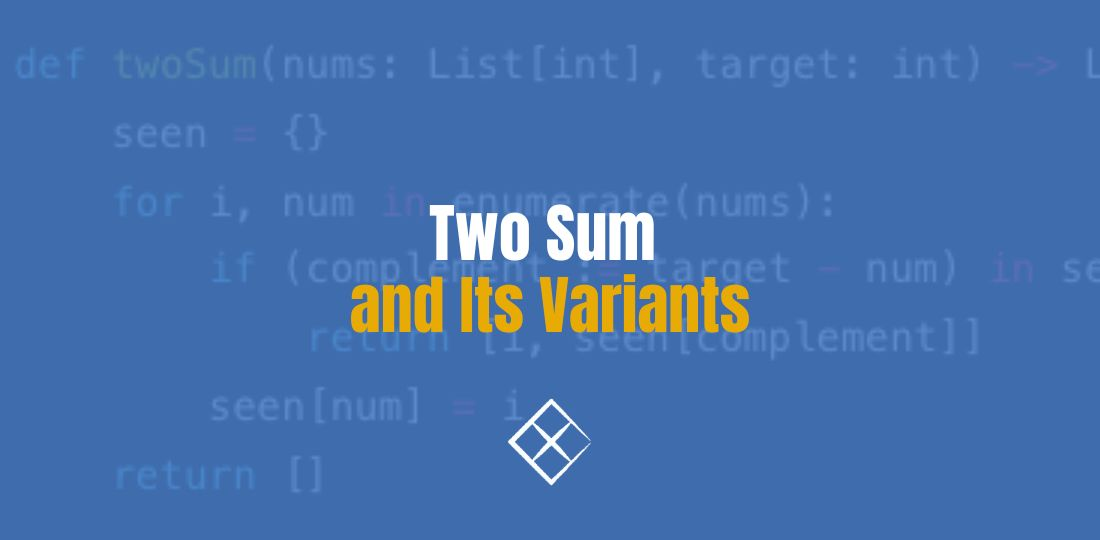# Two Sum and Its Variants# Problem Statement

Given an array of integers `nums` and an integer `target`, return `indices` of the two numbers such that they add up to target.

You may assume that each input would have exactly one solution, and you may not use the same element twice.

You can return the answer in any order.

``````Example 1:

Input: nums = [2,7,11,15], target = 9
Output: [0,1]
Explanation: Because nums + nums == 9, we return [0, 1].

Example 2:

Input: nums = [3,2,4], target = 6
Output: [1,2]

Example 3:
Input: nums = [3,3], target = 6
Output: [0,1]
``````

## Brute Force Solution

A straightforward approach is to try all pairs of indices `(i, j)` and check if `nums[i] + nums[j] == target`. This approacha has quadratic running time and uses constant extra space (not counting the space used by `output`).

## Hash Map Solution

An optimized approach is to store the numbers in a Hash Table which enables constant lookup time:

``````def twoSum(nums: List[int], target: int) -> List[int]:
seen = {}
for i, num in enumerate(nums):
if (complement := target - num) in seen:
return [i, seen[complement]]
seen[num] = i
return []
``````

The time and space complexity is both `O(n)`. Obviously the linear running time is optimal as a linear scan of `nums` is inevitable.

## Variant I: Data Structure Design

``````from collections import Counter

class TwoSum:
def __init__(self):
self.counts = Counter()

def add(self, number: int) -> None:
self.counts[number] += 1

def find(self, value: int) -> bool:
return any(
(complement := value - num) in self.counts and
(complement != num or count > 1)
for num, count in self.counts.items()
)
``````

## Variant II: Input is sorted.

When `nums` is sorted we can use two-pointers technique:

``````def twoSum(numbers: List[int], target: int) -> List[int]:
left, right = 0, len(numbers) - 1
while left < right:
if numbers[left] + numbers[right] < target:
left += 1
elif numbers[left] + numbers[right] > target:
right -= 1
else:
return [left + 1, right + 1]
``````

Time complexity: `O(n)`

Space complexity: `O(1)`

## Variant III: Less Than K

``````def twoSumLessThanK(nums: List[int], k: int) -> int:
nums.sort()
i, j = 0, len(nums) - 1
ans = -1
while i < j:
if (two_sum := nums[i] + nums[j]) >= k:
j -= 1
else:
ans = max(ans, two_sum)
i += 1
return ans
``````

Time complexity: `O(nlogn)`

Space complexity: `O(n)` – Python uses timsort which has worst case linear space complexity.

## Variant IV: Three Sum

``````def threeSum(nums: List[int]) -> List[List[int]]:
nums.sort()
result = []
for i, a in enumerate(nums):
if a > 0:
break
if i > 0 and a == nums[i-1]:
continue
for b, c in two_sum(nums, i+1, -a):
result.append([a, b, c])
return result

def two_sum(nums, start_index, target):
i, j = start_index, len(nums) - 1
while i < j:
x, y = nums[i], nums[j]
if i > start_index and nums[i-1] == x:
i += 1
continue
if x + y == target:
yield x, y
i += 1
j -= 1
elif x + y < target:
i += 1
else:
j -= 1
``````

Time Complexity: `O(n^2)`

Space Complexity: `O(n)`